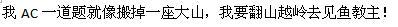# 往届 ACM 队训练题 (参考)

1084. WYI

As eveyone known, WY is the most faithful fan of Lord fishcanfly. The power of Lord fish is too great to reach, but WY decides to train himself to near the Lord as much as he can. He even makes a slogan as follows:So WY wants to AC many problems. But he has only a little time :(, so he cannot solve all of them. He needs the help of other Lord Fish’s fans to make a plan to do most training without exceeding the time limit. Of course, it’s useless to solve the same problem two or more times, because he can only learn knowledge at the first time.

### 输入格式

Input contains multiple test case. The first line is T, the number of test case, then T case follows. Each test case begins with two integers 1 <= N <= 100 and 1 <= L <= 10^9, which denote the number of problems and the time WY can spend at most. The following N lines each represents a problem with two positive integers 1 <= t <= 10^7 and k, means WY must spend t units of time to solve this problem, and he can learn k units of knowledge after solving it. Because of the bad habit of WY, he is interested in solving easy problems. That is to say, he cannot learn much knowledge once. So each k is no greater than 200.

### 输出格式

For each test case, print an integer which is the most number of units of knowledge WY can learn after the training.

### 样例

Input
4
3 100
10 10
20 10
30 10
2 50
60 100
51 90
2 50
40 10
49 11
4 10000000
2500000 1
2500000 1
2500000 1
2500000 1

Output
30
0
11
4